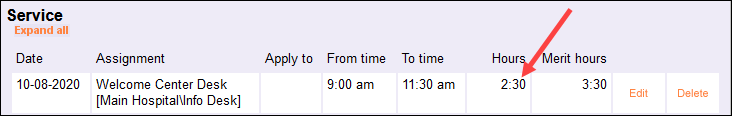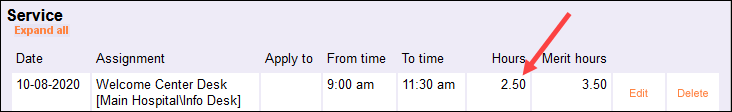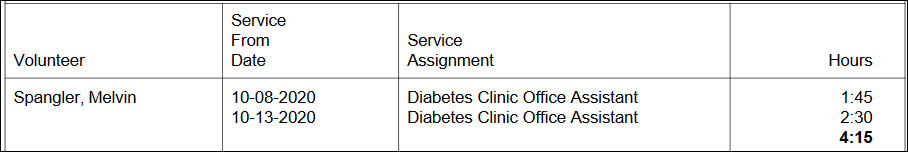# Difference Between Decimal and Hours:Minutes Formats¶

In Volgistics, service hours can be displayed in two different formats. They can be shown in an Hours:Minutes format or in a Decimal format. This help topic will discuss the nuances of these two formatting options, provide some useful tips for each option, and explore their differences.

By default, Volgistics will use the Hours:Minutes format for service entries, but you can change this in your account settings. If you'd like to review or change these settings:

1. Choose Setup from the menu.
2. Expand Service Tracking.
3. Click the Ground Rules link.
4. In the Hour entry display format section, choose your preferred format for volunteer service hours.
5. Click the Save button.

The format you choose for volunteer service will affect how you enter hours and how hours are displayed within your account and on your reports. However, please keep in mind that any report generated in the Excel file format will always display service hours in the Decimal format. Even if you use the Hours:Minutes format in your account, Excel reports will always show service hours in the Decimal format.

With that in mind, let's take a look at each of these formats in more depth.

## Hours:Minutes Format¶

The Hours:Minutes format is the default formatting option used in Volgistics. With this option selected, your volunteer service entries will indicate the total number of hours and minutes the volunteer served, and these two values will be separated by a colon `:`. If you see volunteer service information with a colon, this indicates that those service hours are being displayed in the Hours:Minutes format. For example, if a volunteer served for 2 hours and 30 minutes, this would be entered and displayed as 2:30. Below is an example of what this looks like on the Service tab of a volunteer record.When using this format, it is important to keep in mind that there are only 60 minutes in an hour, so the Minutes value of a service entry will only range from 0 to 59. If a volunteer has served 60 minutes, this would be the same as one hour and would be represented as 1 hour and 0 minutes in Volgistics. Therefore, this would be displayed as 1:00.

Any service over 59 minutes should be reformatted into its equivalent number of hours and minutes. As another example, let's say that a volunteer served for 75 minutes. This is equivalent to 1 hour and 15 minutes of service. Therefore, that service will need to be entered as 1:15.

## Decimal Format¶

The Decimal format for volunteer service entries will indicate the number of hours and partial hours served using a two decimal number. Rather than displaying the number of hours and minutes served as two separate values, the Decimal format provides all of this information in a single number where the minutes are represented as a percentage of an hour.

To explain this further, let's look at an example. Let's say that you have a volunteer who served for 2 hours and 30 minutes. This volunteer served for 2 full hours, but they also served an additional 30 minutes which is only a portion of a full hour. Specifically, 30 minutes is exactly half of a full hour, so in the Decimal format this would be represented as 0.50 hours. Therefore, this volunteer's total service would be entered and displayed as 2.50 hours (2 and a half hours). The image below shows how this looks on the Service tab of a volunteer record.Careful!

Please note that the Decimal format and Hours:Minutes format use different forms of punctuation. The Hours:Minutes format uses a colon `:`, while the Decimal format uses a decimal point `.`. These two kinds of punctuation cannot be used interchangeably when you are recording volunteer service since these represent two different methods of displaying information.

For example, let's say you have a volunteer who served for 3 hours and 45 minutes. In the Hours:Minutes format, this would be recorded as 3:45. However, this is NOT the same as 3.45 hours. Those 45 minutes are actually three-quarters of an hour, which is equal to 0.75 hours. For this reason, this volunteer's service would actually be represented as 3.75 hours in the Decimal format.

So if you are working with the Decimal format, you'll want to make sure that you understand these differences. In the next section, you can learn how to convert between the two formats, and you can find a complete chart showing how to convert Minutes values to Decimal hour values.

## Converting Service Hours Between Formats¶

There may be cases where you need to convert between the two service hour formats. For instance, if you use the Hours:Minutes format within your account but generate a Service report in the Excel format, the service on the report will show in the Decimal format (as mentioned above). In this case, you may want to convert some of the service values on that report back to an Hours:Minutes format.

You can do this conversion manually using a little mathematics. There are 60 minutes in an hour, and each minute is one-sixtieth (1/60) of an hour. We can use this information to convert from one format to the other.

### Converting from Hours:Minutes to Decimal Format¶

Let's start with converting from Hours and Minutes to the Decimal format. The number on the left side of the colon in the Hours:Minutes format represents the number of full hours served, so we already have that information when converting to the Decimal format. Next we'll need to convert the Minutes value to a decimal. Since our decimal value is indicating what percentage of an hour those minutes equate to, we'll calculate this decimal by dividing the number of Minutes by 60. Then once we have that decimal value, we'll simply add it to the number of full hours served.

For example, let's say you have a volunteer who served 4 hours and 39 minutes. We know that the volunteer served for 4 full hours, so now we need to convert the 39 minutes to a decimal. We'll do this by dividing that value by 60:

39 ÷ 60 = 0.65

Those 39 minutes are equal to 0.65 hours. Now we can add that to the 4 full hours of service:

4.00 + 0.65 = 4.65

So 4 hours and 39 minutes (4:39) is equivalent to 4.65 hours in the Decimal format.

### Converting from Decimal Format to Hours:Minutes¶

Next, let's do the opposite; convert from the Decimal format to the Hours:Minutes format. In this case, we also already know the number of full hours served. This is equal to the number on the left side of the decimal point. So our goal is to convert the information on the right side of the decimal point to a Minutes value. The information on the right side of the decimal point indicates what percentage of an hour was served, so to convert this to a Minutes value, we simply multiply it by 60 minutes.

For example, let's say you have a volunteer who served 3.20 hours. We know that the volunteer served for 3 full hours, so now we just need to determine how many minutes is equal to that extra 0.20 hours. We'll do this by multiplying this value by 60 minutes:

0.20 ÷ 60 = 12 minutes

So the 3.20 hours the volunteer served is equivalent to 3 full hours and 12 minutes, or 3:12 in the Hours:Minutes format.

### Conversion Table¶

For simplicity and convenience, you can use the conversion chart below to easily convert between Minutes values and Decimal Hours values:

Minutes Decimal Hours Minutes Decimal Hours
1 0.02 31 0.52
2 0.03 32 0.53
3 0.05 33 0.55
4 0.07 34 0.57
5 0.08 35 0.58
6 0.10 36 0.60
7 0.12 37 0.62
8 0.13 38 0.63
9 0.15 39 0.65
10 0.17 40 0.67
11 0.18 41 0.68
12 0.20 42 0.70
13 0.22 43 0.72
14 0.23 44 0.73
15 0.25 45 0.75
16 0.27 46 0.77
17 0.28 47 0.78
18 0.30 48 0.80
19 0.32 49 0.82
20 0.33 50 0.83
21 0.35 51 0.85
22 0.37 52 0.87
23 0.38 53 0.88
24 0.40 54 0.90
25 0.42 55 0.92
26 0.43 56 0.93
27 0.45 57 0.95
28 0.47 58 0.97
29 0.48 59 0.98
30 0.50 60 1.00

You'll also need to keep in mind which format you're using when you add service hours together. You are certainly familiar with adding decimal numbers together. These numbers can be added together without performing any special procedure or conversion. For example, let's say that you have a volunteer who has served twice with your organization. One time they served 1.75 (1 and three-quarter) hours and another time they served 2.50 (2 and a half) hours. You can add these together in the usual way:

1.75 + 2.50 = 4.25

With this simple math, we can see that this volunteer has served a total of 4.25 (4 and a quarter) hours.

However, things get a little trickier when you're using the Hours:Minutes format. Since these values are not represented as a decimal, they cannot be added like decimals. To investigate this further, let's take a look at the same example as above but using the Hours:Minutes format. From our discussion about converting from the decimal format to the Hours:Minutes format, we know that the two entries the volunteer served are equivalent to 1:45 (1 hour and 45 minutes) and 2:30 (2 hours and 30 minutes) respectively. If we generate a report showing this volunteer's individual service entries and total service, we see something like this:We can see that the volunteer has served a total of 4 hours and 15 minutes (4:15). If we perform our conversion using the information above, we can confirm that this matches up to the 4.25 hours that we calculated when adding the Decimal values together. This is good! But how did Volgistics calculate this using the Hours:Minutes format? Let's walk through that process.

Since these values are not decimal numbers, we'll have to add them together a little differently. Specifically, we'll have to add the hours values and the minutes values together separately. First, we'll add the Minutes values together. The first entry has 45 minutes and the second entry has 30 minutes:

45 + 30 = 75

The minutes portion of these entries adds up to a total of 75 minutes. This is actually more than a full hour and is equivalent to 1 hour and 15 minutes (1:15). So when we move on to add up the Hours values, we'll have to make sure to include the additional 1 hour we got from adding the Minutes and save the remaining 15 minutes for the end.

Now we can add the Hours values. We'll add the 1 hour from the first entry, the 2 hours from the second entry, and the additional 1 hour that resulted from adding the Minutes values together:

1 + 2 + 1 = 4

The total for our Hours value is 4. Putting that together with the remaining Minutes value, we see that the volunteer served a total of 4 hours and 15 minutes (4:15).

Adding these service hours together in the Hours:Minutes format is not quite as straightforward as adding them together as decimals, so you'll need to keep this in mind if you intend to use the Hours:Minutes format.

As an alternative to the process shown here, you could choose to convert your Hours:Minutes value to the decimal format, add those values together, and then convert the total back to the Hours:Minutes format.